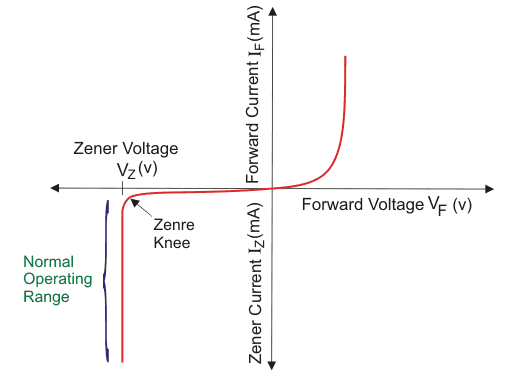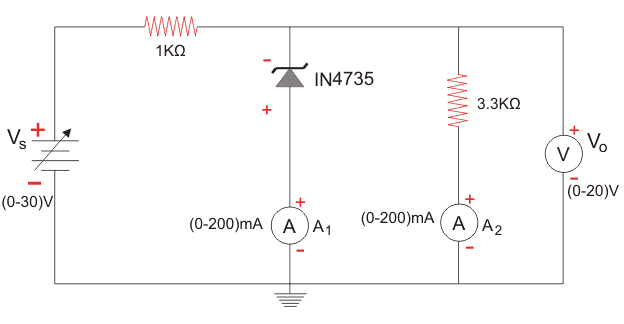# Zener Diode Experiment

The Zener diode mainly operates in reverse biased condition. We use Zener diodes for voltage regulation and voltage stabilisation. They provide a low-cost and no frill method for voltage regulation. The critical parameter of this type of diodes is the Zener breakdown voltage. The Zener breakdown voltage is the minimum reverse biased voltage below which the diode blocks the reverse current through it and above which it causes a significant amount of reverse bias current to flow through it. Once the reverse voltage reaches the Zener breakdown voltage, the voltage across the device remains constant at that level. Hence we can use Zener diode for voltage regulation. The graph of voltage vs current of a diode is called its characteristic. Below you can see the characteristic.Here Vz is the Zener breakdown voltage. We are going to learn about an experiment to find out the Zener breakdown voltage and draw the characteristics of Zener diode. Why should we do this experiment? For correct voltage regulation, we need to find out precisely what voltage should be across the Zener diode. Zener breakdown voltage should be approximately close to the desired voltage we want. Therefore we have to find out the Zener voltage to use that diode appropriately in voltage regulation. For the experiment, we will follow the below circuit diagram.

We need the following equipment:

We need to connect the circuit as given in the diagram above. The experiment is fairly simple to perform. First, we will plot the curve in reverse biased mode. For that, we increase the reverse bias voltage slowly and in small steps. While doing so, keep noting down the ammeter reading and the voltmeter reading. There are two ammeters here, one connected in series with the Zener diode and another one connected in series with the resistance 3.3k Ω. Let’s call the first one as A1 and the second one as A2. If you keep doing so, after a particular value of reverse bias voltage, the value of current in A1 will spike suddenly. Note down the voltmeter reading at this point. The voltmeter reading is the Zener breakdown voltage. Keep increasing the reverse bias voltage further. We will see that the voltage across the diode remains constant whereas the current through it keeps on increasing. Note down the ammeters and voltmeter readings at different values of reverse bias voltage. Tabulate the values of current and voltage.Do the same experiment by connecting the Zener diode in the forward bias. Note down the current and voltage reading.

 Voltage Current

Your table should look something like the one shown above. Populate the values in the table. Then draw the graph corresponding to these values. You will get the characteristic curve of Zener diode. Note down the value at which the current increases rapidly when in reverse biased mode. The voltage at this value is the Zener breakdown voltage. Note down this voltage separately.

Different Zener diodes have a different breakdown voltage. We should select a Zener diode with a breakdown voltage approximately equal to the voltage we need across the device. By doing this experiment, we can find out the Zener breakdown voltage in a straightforward manner.

Want To Learn Faster? 🎓
Get electrical articles delivered to your inbox every week.
No credit card required—it’s 100% free.